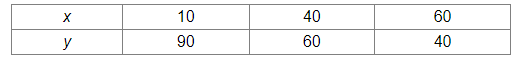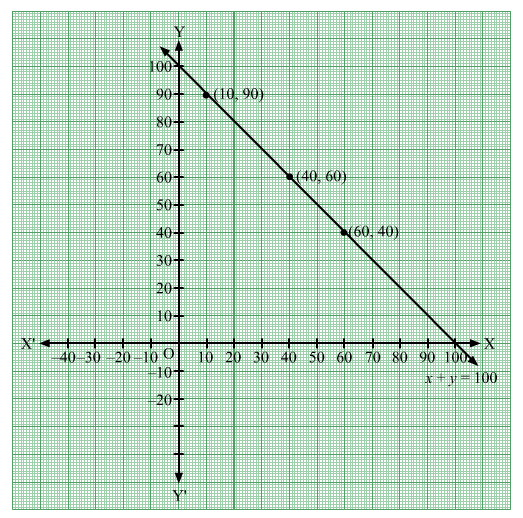# Two students A and B contributed ₹ 100 towards the Prime Minister's Relief Fund to help the earthquake victims

Question:

Two students A and B contributed ₹ 100 towards the Prime Minister's Relief Fund to help the earthquake victims. Write a linear equation to satisfy the above data and draw its graph.

Solution:

Let the contribution of A and B be ₹ and ₹ y, respectively.

Total contribution of A and B = ₹ + ₹ = ₹ (y)

It is given that the total contribution of A and B is ₹ 100.

∴ = 100

This is the linear equation satisfying the the given data.

= 100

⇒ y = 100 – x

When x = 10, y = 100 – 10 = 90

When x = 40, y = 100 – 40 = 60

When x = 60, y = 100 – 60 = 40

Thus, the points on the line = 100  are as given in the following table:Plotting the points (10, 90), (40, 60) and (6040) and drawing a line passing through these points, we obtain the graph of of the line = 100.